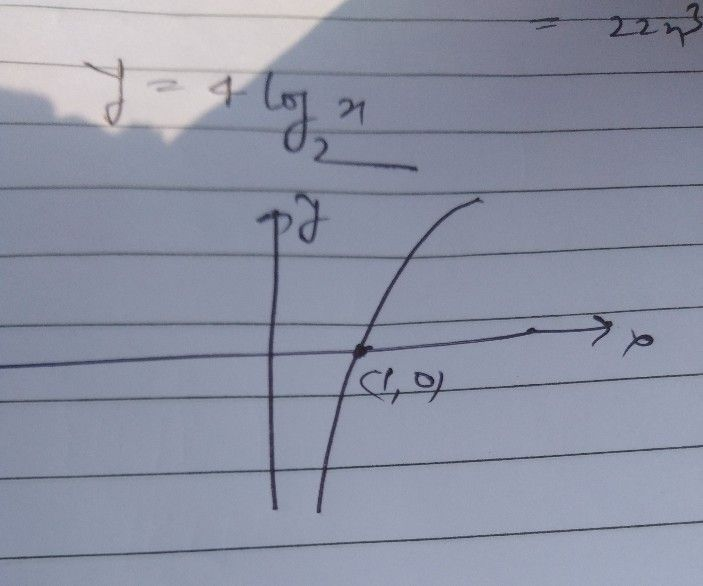Symbol
ProblemWeek 8 Task $5$ For each of the following functions; (a) use transformations to describe how the graph is related to a logarithmic function $=$ $g3$ (b) sketch the graph; (c) identify the domain, range, vertical asymptote, x- intercept, and $0$ $\left($ $57$ $cx033$ $37=o3$ $60\right)$
10th-13th grade
Other
Search count: 106
SolutionQanda teacher - jaisiStudent
sir can you illustrate further because I can't understand